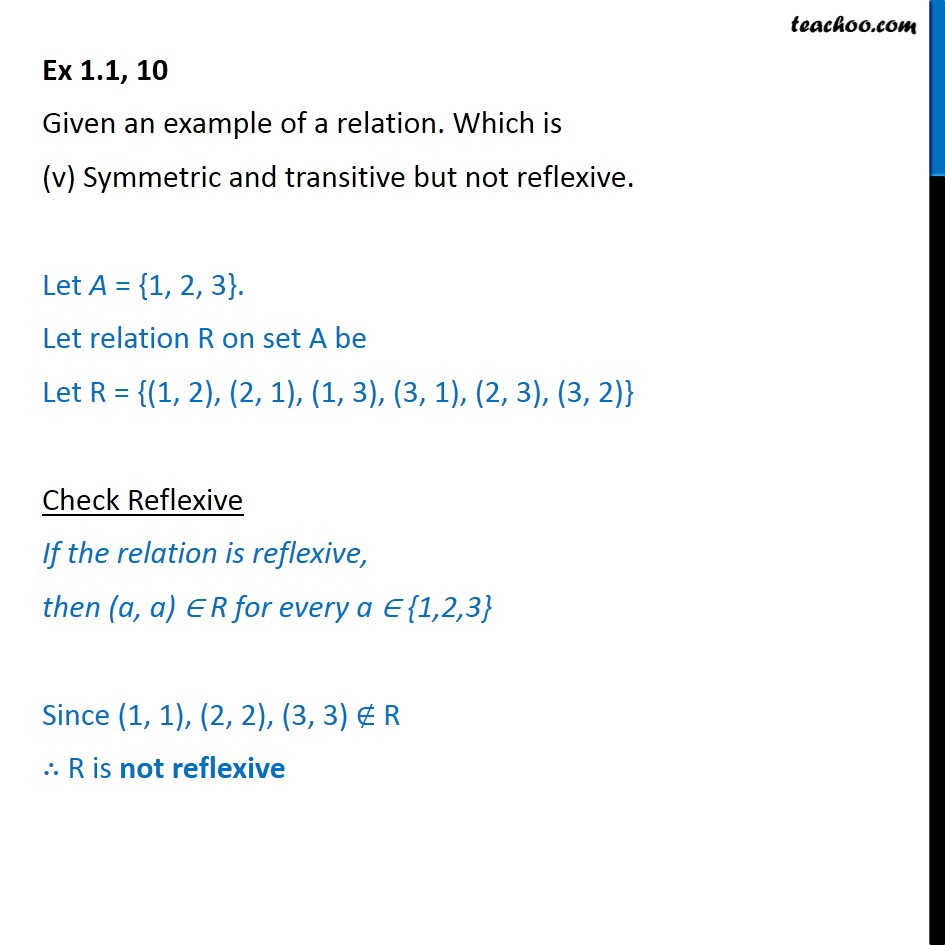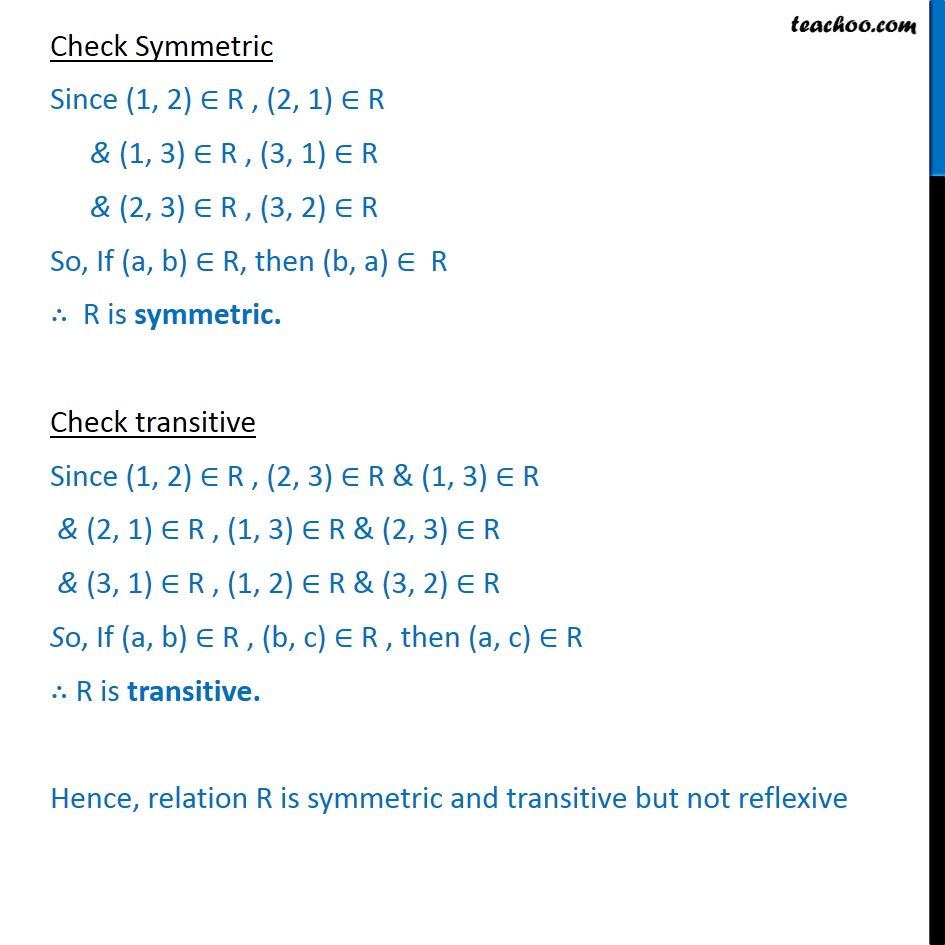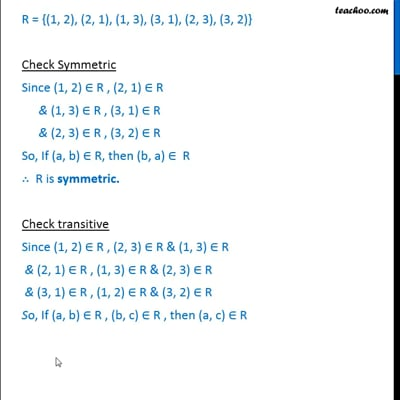Ex 1.1

Chapter 1 Class 12 Relation and Functions
Serial order wiseThis video is only available for Teachoo black users

Maths Crash Course - Live lectures + all videos + Real time Doubt solving!

### Transcript

Ex 1.1, 10 Given an example of a relation. Which is (v) Symmetric and transitive but not reflexive. Let A = {1, 2, 3}. Let relation R on set A be Let R = {(1, 2), (2, 1), (1, 3), (3, 1), (2, 3), (3, 2)} Check Reflexive If the relation is reflexive, then (a, a) R for every a {1,2,3} Since (1, 1), (2, 2), (3, 3) R R is not reflexive Check Symmetric Since (1, 2) R , (2, 1) R & (1, 3) R , (3, 1) R & (2, 3) R , (3, 2) R So, If (a, b) R, then (b, a) R R is symmetric. Check transitive Since (1, 2) R , (2, 3) R & (1, 3) R & (2, 1) R , (1, 3) R & (2, 3) R & (3, 1) R , (1, 2) R & (3, 2) R So, If (a, b) R , (b, c) R , then (a, c) R R is transitive. Hence, relation R is symmetric and transitive but not reflexive